# Matrix

(Redirected from Matrix multiplication)

A matrix (plural "matrices") is a mathematical construct generally represented as a rectangular array of elements. These elements are usually numbers, but can be other matrices, and other mathematical structures. Matrices with real elements are called "real matrices", matrices with complex elements are called "complex matrices", and so on. Matrices with matrices as elements are called "partitioned matrices".

Among their other applications, matrices are fundamental to the study of linear algebra, since every linear transformation can be represented by a unique matrix, and every matrix represents a unique linear transformation.

##  Dimensions and coordinates

A matrix with m rows and n columns is described as an m×n (pronounced "m by n") matrix, with the number of rows always coming first. When one dimension of a matrix is equal to 1 -- that is, in the case of a 1×n or m×1 matrix -- the matrix is a vector. A matrix with one row is a row vector; a matrix with one column is a column vector.

If the m×n matrix is named A, individual entries are named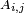$A_{i,j}$, where$\scriptstyle1 \leq i \leq m$ and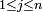$1 \leq j \leq n$; again, the row coordinate comes first. For example, suppose M is a 3×4 matrix:$M = \begin{pmatrix} 7 & 4.3 & 9 & -3 \\ 0 & 6 & 18 & 42 \\ -10 & 9.5 & 16 & 0 \end{pmatrix}$

Now we can say that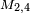$M_{2,4}$ = 42: the element in the second row and the fourth column, counting from the top left.

Notational conventions vary; the comma in the subscript is sometimes omitted, so the same entry would be named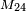$M_{24}$; of course, this notation is only practical when the matrix in question is smaller than 10×10. A superscript-subscript notation is sometimes used, where the row coordinate appears as a superscript and the column coordinate appears as a subscript, thus:$M^2_4 = 42$. While upper-case letters are almost universally used for matrices themselves, some texts maintain the upper-case letter for the individual elements (e.g.$M_{2,4}$) while others use lower-case (e.g.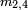$m_{2,4}$). Finally, in typesetting the matrix itself, some texts place large parentheses around the elements while others use large square brackets.

##  Operations

Several operations are defined for matrices.

Two matrices may be added if and only if they have identical dimensions. The sum of the matrices is simply the matrix composed of the sums of the entries. That is, if A and B are m×n matrices, then A + B is an m×n matrix such that$(A + B)_{i,j} = A_{i,j} + B_{i,j}$ for all i, j with$1 \leq i \leq m$ and$1 \leq j \leq n$

For example:$\begin{pmatrix} 1 & 2 & 3 \\ 4 & 5 & 6 \end{pmatrix} + \begin{pmatrix} 9 & 16 & 12 \\ 5 & 17 & 15 \end{pmatrix} = \begin{pmatrix} (1 + 9) & (2 + 16) & (3 + 12) \\ (4 + 5) & (5 + 17) & (6 + 15) \end{pmatrix} = \begin{pmatrix} 10 & 18 & 15 \\ 9 & 22 & 21 \end{pmatrix}$

A + B = B + A

and associative:

A + (B + C) = (A + B) + C

###  Scalar multiplication

Any scalar may be multiplied by any matrix. To obtain the resultant matrix, multiply each entry of the original matrix by the scalar. That is, if c is a scalar and A is an m×n matrix, then cA is an m×n matrix such that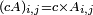$(cA)_{i,j} = c \times A_{i,j}$

For example:$3 \begin{pmatrix} 1 & 2 & 3 \\ 4 & 5 & 6 \end{pmatrix} = \begin{pmatrix} (3 \times 1) & (3 \times 2) & (3 \times 3) \\ (3 \times 4) & (3 \times 5) & (3 \times 6) \end{pmatrix} = \begin{pmatrix} 3 & 6 & 9 \\ 12 & 15 & 18 \end{pmatrix}$

###  Matrix multiplication

Two matrices A and B may be multiplied if A has as many columns as B has rows. (Otherwise, they are said to be incompatible and their product is undefined.) That is, an m×n matrix may be multiplied by an n×p matrix. Then the resultant matrix AB is m×p and the (i,j)th entry of AB is the vector dot product of the ith row of A and the jth column of B. Formally: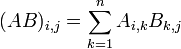$(AB)_{i,j} = \sum_{k=1}^n A_{i,k} B_{k,j}$

For example: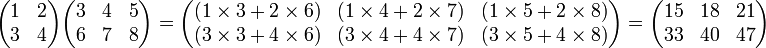$\begin{pmatrix} 1 & 2 \\ 3 & 4 \end{pmatrix} \begin{pmatrix} 3 & 4 & 5 \\ 6 & 7 & 8 \end{pmatrix} = \begin{pmatrix} (1 \times 3 + 2 \times 6) & (1 \times 4 + 2 \times 7) & (1 \times 5 + 2 \times 8) \\ (3 \times 3 + 4 \times 6) & (3 \times 4 + 4 \times 7) & (3 \times 5 + 4 \times 8) \end{pmatrix} = \begin{pmatrix} 15 & 18 & 21 \\ 33 & 40 & 47 \end{pmatrix}$

Even if AB is defined, BA may not be. If both matrices are defined, they may have different dimensions. Even if they have the same dimensions, they may not be equal. Thus, matrix multiplication is clearly not commutative. It is, however, associative:

A(BC) = (AB)C

and left- and right-distributive:

A(B + C) = AB + AC and (A + B)C = AC + BC

so long as all the relevant products are defined.

###  Transposition

Given an m×n matrix A, its transpose (denoted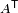$A^\mathsf{T}$) is an n×m matrix where each row in A is a column in$A^\mathsf{T}$ and vice versa. That is: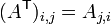$(A^\mathsf{T})_{i,j} = A_{j,i}$

For example: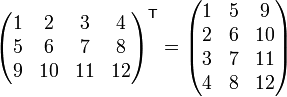$\begin{pmatrix} 1 & 2 & 3 & 4 \\ 5 & 6 & 7 & 8 \\ 9 & 10 & 11 & 12 \end{pmatrix}^{\mathsf{T}} = \begin{pmatrix} 1 & 5 & 9 \\ 2 & 6 & 10 \\ 3 & 7 & 11 \\ 4 & 8 & 12 \end{pmatrix}$

Note that the transpose operation is its own inverse; for all matrices A,$(A^\mathsf{T})^{\mathsf{T}} = A$.

##  Special matrices

Certain types of matrices prove useful in different contexts.

###  Square matrices

A square matrix, as the term implies, is any matrix of dimension n×n -- that is, with the same number of rows as columns. Two n×n matrices may always be multiplied, and their product is another n×n matrix.

###  Identity matrix

We denote by$\mathbb{I}_n$ the multiplicative identity for matrix multiplication; that is, the matrix such that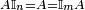$A \mathbb{I}_n = A = \mathbb{I}_m A$ for any m×n matrix A.$\mathbb{I}_n$ takes the form of a n×n square matrix with the number 1 down its main diagonal, starting from element (1, 1), and the number 0 everywhere else. So$\mathbb{I}_1 = \begin{pmatrix}1\end{pmatrix}$,$\mathbb{I}_2 = \begin{pmatrix} 1 & 0 \\ 0 & 1 \end{pmatrix}$,$\mathbb{I}_3 = \begin{pmatrix} 1 & 0 & 0 \\ 0 & 1 & 0 \\ 0 & 0 & 1 \end{pmatrix}$, ...

In general, the n subscript is included only if necessary; if the size of the identity matrix can be deduced from context, we omit the subscript. For example, we would most likely say:$A \mathbb{I} = A = \mathbb{I} A$

since only one identity matrix is dimensionally compatible for multiplication.

###  Zero matrix

The additive identity under matrix addition is known as a zero matrix, and denoted$0_{m,n}$ for an m×n matrix. Its entries are all zeroes, so (for example)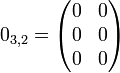$0_{3,2} = \begin{pmatrix} 0 & 0 \\ 0 & 0 \\ 0 & 0 \end{pmatrix}$

It is evident that for any m×n matrix A,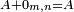$A + 0_{m,n} = A$

It is also clear that the product of any matrix with a zero matrix is another zero matrix, which may or may not have the same dimensions. As with the identity matrix, the subscript is omitted if the context admits only one zero matrix. In this example, any other zero matrix could not be added to A, so the subscript is redundant and we could say

A + 0 = A

###  Invertible matrix

Some, but not all, matrices have a multiplicative inverse. That is, for a matrix A, there may exist a matrix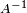$A^{-1}$ such that$A A^{-1} = A^{-1} A = \mathbb{I}$

Only square matrices may be inverted. Furthermore, if the determinant of a square matrix is 0, it is singular -- that is, not invertible.

Some square matrices without inverses may have matrix "pseudoinverses", which have properties extending the concept inverses.

###  Symmetric matrix

A symmetric matrix is equal to its transpose. It must therefore be a square matrix, with values reflected across the main diagonal. That is, if A is an n×n matrix, A is symmetric if and only if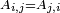$A_{i,j} = A_{j,i}$ for all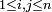$1 \leq i, j \leq n$

For example:$A = \begin{pmatrix} 1 & 2 & 3 & 4 \\ 2 & 5 & 6 & 7 \\ 3 & 6 & 8 & 9 \\ 4 & 7 & 9 & 10 \end{pmatrix} = A^\mathsf{T}$

###  Antisymmetric matrix

An antisymmetric or skew-symmetric matrix is the additive inverse of its transpose. It must also therefore be a square matrix. If A is an n×n matrix, A is antisymmetric if and only if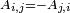$A_{i,j} = -A_{j,i}$ for all$1 \leq i, j \leq n$

Therefore, it is a requirement that all entries on the main diagonal of an antisymmetric matrix equal zero. For example:$A = \begin{pmatrix} 0 & 1 & 2 & 3 \\ -1 & 0 & 4 & 5 \\ -2 & -4 & 0 & 6 \\ -3 & -5 & -6 & 0 \end{pmatrix} \Longrightarrow A^{\mathsf{T}} = \begin{pmatrix} 0 & -1 & -2 & -3 \\ 1 & 0 & -4 & -5 \\ 2 & 4 & 0 & -6 \\ 3 & 5 & 6 & 0 \end{pmatrix}$

###  Diagonal matrix

A matrix that has zeros everywhere other than the main (upper left to lower right) diagonal is a Diagonal matrix.

Example: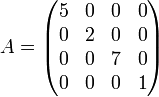$A = \begin{pmatrix} 5 & 0 & 0 & 0 \\ 0 & 2 & 0 & 0 \\ 0 & 0 & 7 & 0 \\ 0 & 0 & 0 & 1 \end{pmatrix}$

Diagonal matrices are especially easy to invert or operate with.

###  Scalar matrix

A diagonal matrix all of whose diagonal entries are equal is a Scalar matrix. Scalar matrices are so called because their multiplicative action on other matrices is the same as multiplication by a scalar element. A scalar matrix is just a scalar multiple of the identity matrix.

Example: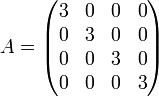$A = \begin{pmatrix} 3 & 0 & 0 & 0 \\ 0 & 3 & 0 & 0 \\ 0 & 0 & 3 & 0 \\ 0 & 0 & 0 & 3 \end{pmatrix}$

###  Triangular matrix

A matrix that has only zeros above (below) the main diagonal is called a lower (upper) triangular matrix. Examples:

Upper triangular: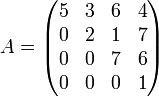$A = \begin{pmatrix} 5 & 3 & 6 & 4 \\ 0 & 2 & 1 & 7 \\ 0 & 0 & 7 & 6 \\ 0 & 0 & 0 & 1 \end{pmatrix}$

Lower triangular: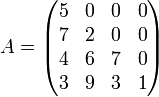$A = \begin{pmatrix} 5 & 0 & 0 & 0 \\ 7 & 2 & 0 & 0 \\ 4 & 6 & 7 & 0 \\ 3 & 9 & 3 & 1 \end{pmatrix}$

Triangular matrices are especially easy to invert or operate with.

###  Orthogonal matrix

A matrix is Orthogonal if the transpose of the matrix is equal to its inverse.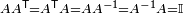$A A^\mathsf{T} = A^\mathsf{T} A = A A^{-1} = A^{-1} A = \mathbb{I}$

###  Hermitian Matrix

A Hermitian matrix (or self-adjoint matrix) is one which is equal to its Hermitian adjoint (also known as its conjugate transpose). That is to say that every entry in the transposed matrix is replaced by its complex conjugate:$a_{i,j}=\overline{a_{j,i}}$,
or in matrix notation: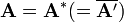$\mathbf{A=A^*(=\overline{A'})}$

###  Idempotent Matrix

A matrix is Idempotent if it is equal to its square.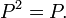$P^2 = P .\,$

An idempotent matrix P has eigenvalues 0 or 1 and has a basis of eigenvectors: it is diagonalisable since its minimal polynomial polynomial X2-X has no repeated roots. The kernel and image of P are complements: they form an internal direct sum.

###  Sparse Matrix

If a matrix has enough zero elements that one can take advantage of the fact, a matrix is called a "Sparse Matrix". Triangular matrices and Diagonal matrices are examples of sparse matrices

##  Applications

###  Systems of linear equations

Matrix techniques are often used to solve systems of equations in several variables, because any system of linear equations may be represented in matrix form. For example, the system

a1x + b1y + c1z = d1
a2x + b2y + c2z = d2
a3x + b3y + c3z = d3

is equivalent to the equation$\begin{pmatrix} a_1 & b_1 & c_1 \\ a_2 & b_2 & c_2 \\ a_3 & b_3 & c_3 \\ \end{pmatrix} \begin{pmatrix} x \\ y \\ z \end{pmatrix} = \begin{pmatrix} d_1 \\ d_2 \\ d_3 \end{pmatrix}$

where the unknowns are entirely within the second matrix. Then, if the first matrix is invertible, x, y, and z can be recovered: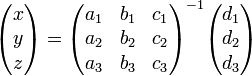$\begin{pmatrix} x \\ y \\ z \end{pmatrix} = \begin{pmatrix} a_1 & b_1 & c_1 \\ a_2 & b_2 & c_2 \\ a_3 & b_3 & c_3 \\ \end{pmatrix}^{-1} \begin{pmatrix} d_1 \\ d_2 \\ d_3 \end{pmatrix}$

Solving linear systems is extensively in Physics, Mechanics, and many other fields.

###  Linear transformations

If f is a linear mapping from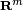$\mathbf{R}^m$ to$\mathbf{R}^n$, then there exists a unique m×n matrix F such that for any vector x in$\mathbf{R}^m$,$f(\mathbf{x}) = F \mathbf{x}$

These transformations have extensive use in Computer Graphics, and in mechanics.

###  Least Squares problems

Surveying problems with redundant (and not quite consistant) measurements were first studied by Gauss and he developed "Least Squares" to find solutions that were "close" to the systems the data described. The exact sense of "close" was that the solution had the lowest possible sum of the squares of the differences between the data at hand and the solution given. He pioneered the use of matrix "Normal Equations" for the problem, although today matrix "Orthogonalization" methods such as QR factorization are more commonly used.

###  Finite Element methods

Breaking an object into a mesh of points, and computing the interactions of those points, is used in a number of Engineering fields to model materials. Various matrix methods are used to deal with the problem.Some content on this page may previously have appeared on Citizendium.